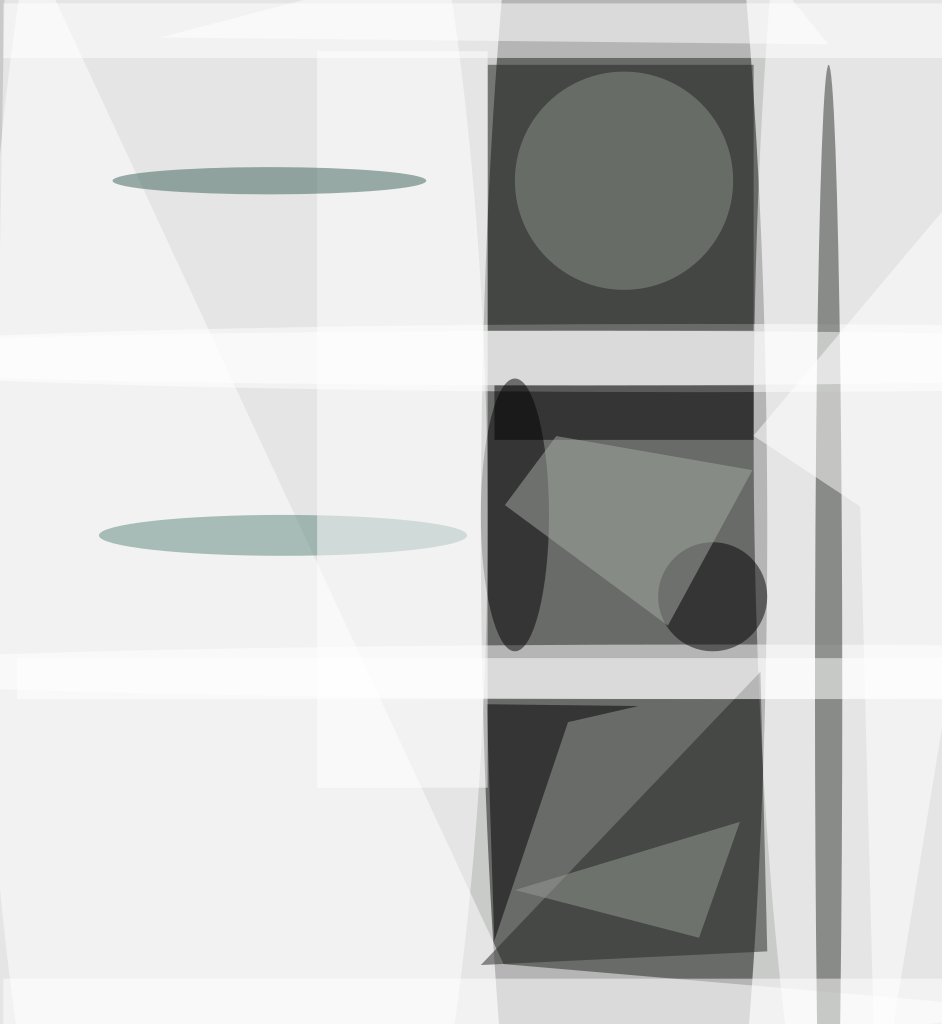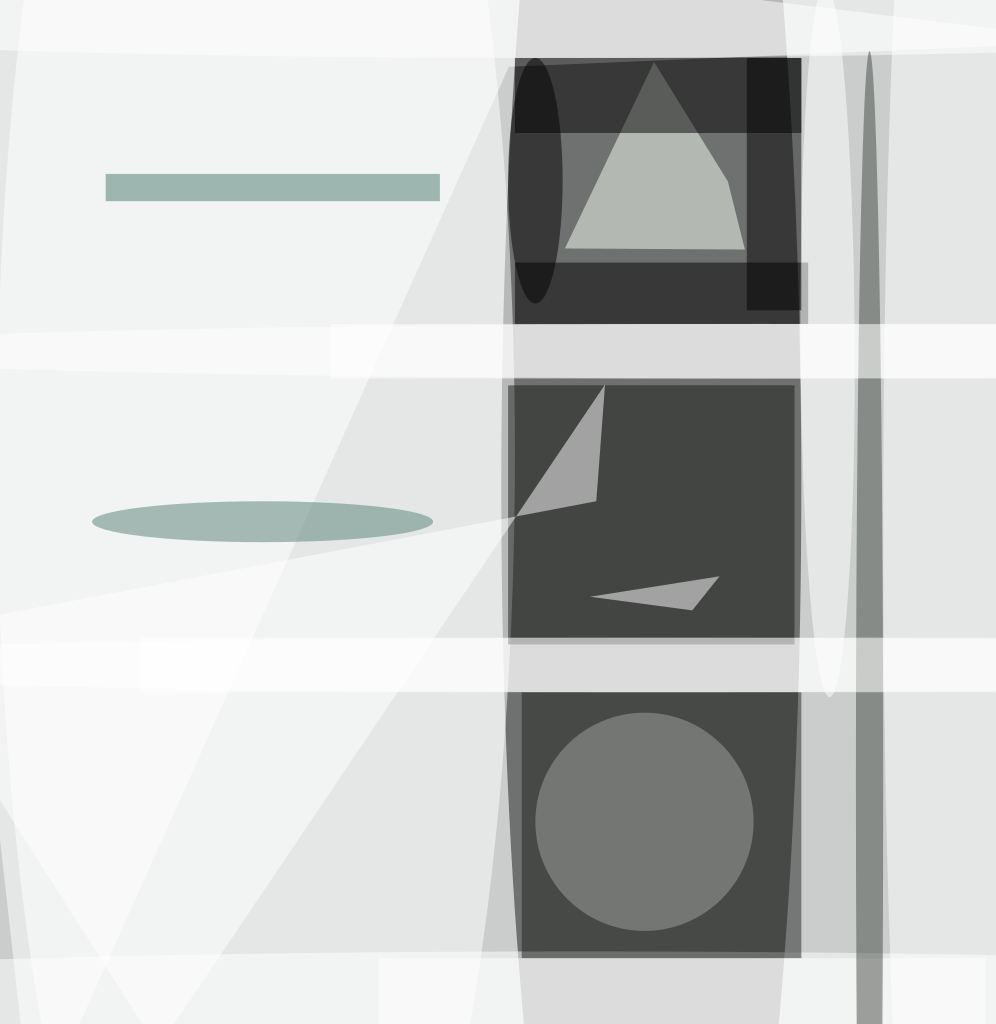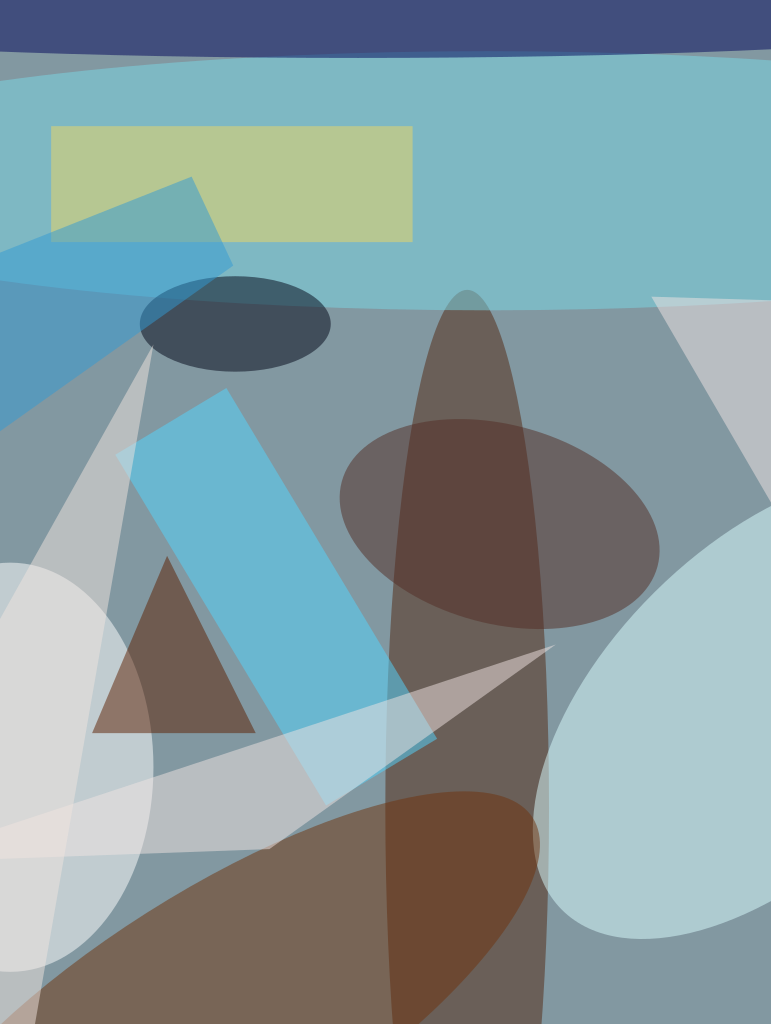An analysis of the classical method of calculating the zero-gravity surface figure of a mirror from surface-figure measurements in the presence of gravity has led to improved understanding of conditions under which the calculations are valid. In this method, one measures the surface figure in two or more gravity-reversed configurations, then calculates the zero-gravity surface figure as the average of the surface figures determined from these measurements. It is now understood that gravity reversal is not, by itself, sufficient to ensure validity of the calculations: It is also necessary to reverse mounting forces, for which purpose one must ensure that mounting-fixture/mirror contacts are located either at the same places or else sufficiently close to the same places in both gravity-reversed configurations. It is usually not practical to locate the contacts at the same places, raising the question of how close is sufficiently close. The criterion for sufficient closeness is embodied in the St. Venant principle, which, in the present context, translates to a requirement that the distance between corresponding gravity-reversed mounting positions be small in comparison to their distances to the optical surface of the mirror.Fig. 1. FEA Modeling of the Surface Figure of PT-M1 during gravity reversal in a simple mirror mount in which the mirror rests on 3 points of contact near the rim (cartoons at left). The mirror model is specified to have a spherical surface in the absence of applied forces. The “face-up” and “face-down” orientations experience gravity forces that are reversed. However, mount forces in the two cases are applied at positions separated by the thickness of the mirror rim, so are only imperfectly reversed. Deformations in the two configurations are shown in the top two panels; their average is shown in the bottom panel, which recovers the ideal spherical surface (which would look flat in this display of departure from sphericity) marred by some dimple artifacts near the rim. The rms error in the average is 11.5 nm. The necessity of reversing mount forces is apparent in the behavior of the equations familiar from finite element analysis (FEA) that govern deformation of the mirror. In FEA, the three-dimensional solid body (mirror) is approximated by a mesh of N points, and positions of these points, or nodes, are represented by a 3N-dimensional coordinate vector xi. In the absence of forces, node positions are described by the zero-gravity position vector xi0g that we wish to extract. Forces, also represented by a 3N-dimensional vector fi, cause deviations δxi from the zero-gravity mirror shape; the case of interest is normal gravity (1g), for which we may write the altered elemental positions as

xi1g= xi0g + δxi (1)

These forces may be either body forces due to gravity (fg) or boundary forces due to the mirror mount (fm). The displacements may then be found from

Aijδxj=fi = fig + fim (2)

where paired indices are summed over in the usual convention, and Aij is the “stiffness matrix”. The stiffness matrix is generally sparse, so that a given node is significantly affected only by a small number of nearby nodes; with suitable numbering, it will be nearly diagonal. In the mirror frame, the stiffness matrix does not change when the mirror is rotated among orientations. If the mirror is rotated into a new orientation in which body forces due to gravity reverse direction, and assuming that mount forces reverse as well, the new set of surface displacements δxi' will obey

Aijxj'=fi' = –fig – fim (3)

in the frame of the mirror. Comparing to Equation (2) shows that

δxj'= –δxj for all j (4)

In other words, the average of the deviations from the ideal zero-gravity surface in the two orientations is zero, so the average figure is just the zero-gravity surface. Algebraically, this property may

be expressed as

1/2(xi1g + xi1g')= 1/2(xi0g + δxi + xi0g + δxi')= xi0g (5)Fig. 2. FEA Modeling of the Surface Figure of PT-M1 during gravity reversal in an improved scheme incorporating good mount force reversal. The mount now consists of 3 point contacts bonded to the hub at the back of the mirror (cartoons at left); mount forces thus reverse and are applied at very nearly the same positions in the two orientations, far from the mirror surface. As a result, the surface figures (top two panels at right) are nearly exactly complementary, resulting in an average map (lower right panel) with a formal rms error of only 0.0003 nm. If forces do not reverse perfectly, localized surface artifacts or “dimples” will be seen on the zero-gravity mirror map. Precise gravity reversal is relatively easy to achieve, but some care must be taken to ensure that mount forces, fim, also reverse. Furthermore, the reversed mount forces must be applied at the same points i of the mirror. The tolerance on placing mount forces in the two configurations is set by the St. Venant principle, which captures the basic annealing or space-averaging property of the elliptic partial differential equations governing solid-body deformations in the usual elastic, small-deformation regime. Remarkably, in practice, small position errors in force location are insignificant at distances through the glass of perhaps only 1.5 times their value.

A simple illustration of these principles is provided by the problem of mounting the PT-M1 mirror for zero-gravity surface figure testing. This mirror is a spherical prototype of the largest mirror of the SIM compressor in its former TMA (three-mirror anastigmat) design. The PT-M1 mirror measures about 343 mm in diameter, has a radius of curvature of about 2.2 m, and a surface quality spec of 6.3 nm rms (λ/100) under zero-gravity conditions. It has an areal density of 41.9 km-m–2. This demanding surface spec, coupled with aggressive lightweighting, makes precise attention to mounting schemes critical during measurement of the zero-gravity surface if dimple artifacts are to be avoided. A rudimentary mounting scheme for “face-up/face-down” measurements whose average will yield the zero-gravity surface is shown in Figure 1. Support against gravity is provided from beneath the rim of the mirror, at two slightly different positions in the two configurations, and the result in the averaged surface map is dimpling at the position of the mounting points. The attendant measurement error (11.5 nm rms) exceeds the mirror spec.

A simple improvement to the mounting scheme is shown in Figure 2. Mount members are now attached by bonding, so mount forces in the two configurations are applied at identical positions. Also, mount members are attached to the hub of the mirror, well away from the mirror surface whose zero-gravity figure is being measured; by the St. Venant principle, small errors in effective positioning of mount members have little effect when propagated to the mirror surface. The resulting error in the zero-gravity averaged map is now only 0.0003 nm rms.

The principles described here represent an explicit clarification and deeper understanding of a classical technique for extracting the zero-gravity surface figure of a mirror from measurements of multiple mounting configurations in normal gravity. While FEA computations are used to analyze particular candidate sets of mount configurations, these principles allow model-free insight into new configurations that are likely to be useful. Additional information and extensions of the particular mounting schemes presented here, including one that offers dramatically improved zero-gravity map fidelity without the need for bonding, are discussed in Bloemhof, Lam, Feria, and Chang, Appl. Opt. Vol. 46, No. 31, p. 7670 (2007).

This work was done by Eric E. Bloemhof of Caltech for NASA’s Jet Propulsion Laboratory. For more information, contact This email address is being protected from spambots. You need JavaScript enabled to view it.##### Photonics Tech Briefs Magazine

This article first appeared in the October, 2010 issue of Photonics Tech Briefs Magazine.

Read more articles from this issue here.

Read more articles from the archives here.